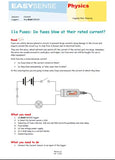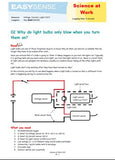# Current - 10A

datalogging sensors

3167

### +/- 10A high range current sensor for d.c. and low voltage a.c. measurements.

1-minute Data Harvest capacitor - charge & discharge

Use in conjunction with voltage sensors to investigate Ohm’s law, power and circuits. This high range sensor is very good for measuring start up current in incandescent, filament lamps and for studying fuses. For smaller currents use the 100 mA or 1A sensors.Teaching applications:
• What changes the current in a circuit, e.g when using light bulbs?
• Can you use bulb brightness to measure current?
• Ohm’s law
• Series and parallel circuits
• Start up current of a lamp• Electrical component characteristics e.g. a light dependent resistor
• Capacitor discharge, charge and energy stored
• Power
• Heat and electric current
• Effect of current on Electromagnet strength Specific Heat Capacity for a liquid or solid

Sensor Ranges:

Range Name Value Resolution Accuracy
Current 100mA ±100mA 50 µA

### Contents/Details:

±100 mA (Resolution 50 µA)
Maximum Voltage ±27 V
Resistance/Impedance OR8 (1.8 Ohms)
• Offset removed by Tare function in software.
• Be aware that some power supplies are ½ wave rectified producing an average rather than true DC. The Current Sensor will ‘pick up’ the fluctuations in voltage and current from this type of power supply.
• The Current sensor should be placed in series with the circuit component through which the current is measured. Currents in either direction can be measured. The Current sensors have a very low resistance so that they introduce as little resistance as possible to the circuit.Fuses (Physics (11-14) eBook)
This activity involves pupils thinking, in detail, about how fuses are used in a circuit as a safety device. It makes use of the Current sensor to measure the current at which a fuse fails.

Fuses overcurrent (Physics (11-14) eBook)
This activity involves pupils thinking, in detail, about how fuses are used in a circuit as a safety device. How quickly does a fuse blow?

Current vs, magnetic field. (Physics (11-14) eBook)
A fixed size coil has current is passed through it. The induced magnetic field is measured for each current. The relationship between current and magnetic field strength can be studied.

Magnetic field and the number of turns in coil. (Physics (11-14) eBook)
A simple coils is used to measure the magnetic field created by a fixed current. The effect of the variable of number of turns is studied.

Electromagnets and alternating current (Physics (11-14) eBook)
how does an electromagnet work if it uses a.c. as the power source?

Specific heat capacity of a liquid (Physics (14-18) : Electricity & Heat eBook)
This investigation will measure the specific heat capacity of a liquid (water). A sample of water of known mass is heated by known number of degrees by an electrical heater.

Stefan - Boltzmann law. (Physics (14-18) : Electricity & Heat eBook)
The experiment illustrates the Stefan - Boltzmann law. It does not give a value for the Boltzmann constant. A simple tungsten filament automotive lamp is used as the source of thermal radiation. The investigation tests if the radiation is really to the 4th power of temperature.

Calibration of a thermometer (Seebeck effect) (Physics (14-18) : Electricity & Heat eBook)
In the investigation two junctions are used, one is placed in a constantly cold temperature the other has varying temperatures applied to it.

Specific heat capacity of a solid (Physics (14-18) : Electricity & Heat eBook)
This investigation will measure the specific heat capacity of a solid. A block of metal of known mass is heated by an electrical heater through a known number of degrees. The electrical energy required to create this temperature rise is determined and used to find the specific heat capacity.

Efficiency of an electric motor / generator (Physics (14-18) : Electricity & Heat eBook)
The efficiency of a motor / generator is determined. This worksheets has details of the motor and generator calculations and method

Efficiency of an electric generator (Physics (14-18) : Electricity & Heat eBook)
Determines the efficiency of a generator. Uses a falling mass as the energy source and records voltage and current to produce power curves.

Efficiency of an electric motor (Physics (14-18) : Electricity & Heat eBook)
uses a known mass lifted through a known distance compared to the power required by a motor to lift the mass.

How does the magnetic field strength vary with current? (Physics (14-18) : Electricity & Heat eBook)
Uses an ac power supply to provide a constantly changing current Using the ac power supply gives multiple repeats of the same experiment .

Force on conductor in a magnetic field (BIL) (Physics (14-18) : Electricity & Heat eBook)
If a conducting wire is placed in a magnetic field and a current is passed, the wire will tend to move in a direction determined by Fleming’s left hand rule. The force making the wire move will be proportional to the current flow and the strength of the magnetic field.Why do bulbs blow (Science At Work (11-16) eBook)
This activity uses this familiar event to introduce the idea of power surges and to calculate resistance changes. It is a practical introduction to the relationship between voltage and current and the problem of correctly fusing devices in a home for safety.

HK\$ 550.00

3167